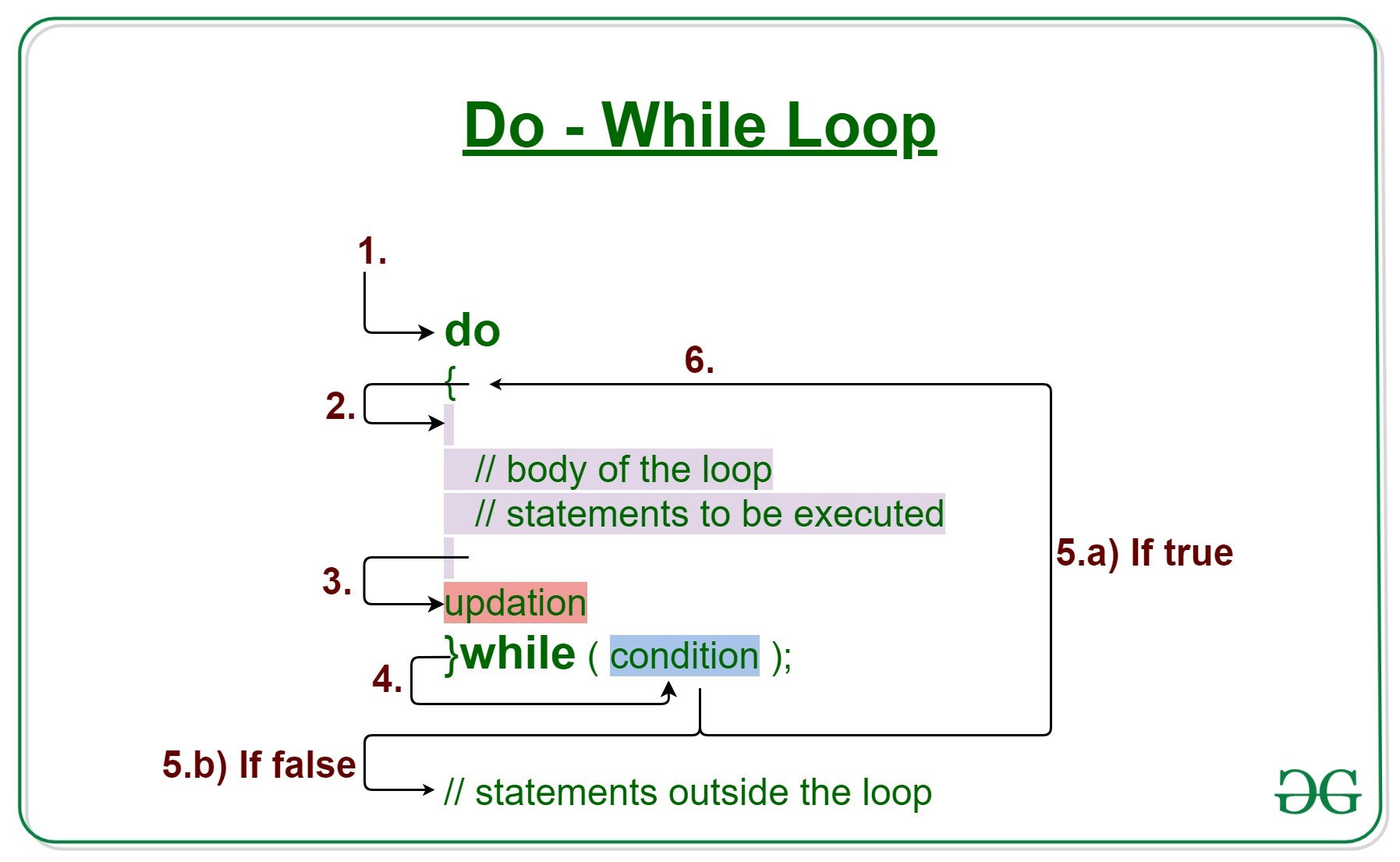# C/C++ do while loop with Examples

Loops in C/C++ come into use when we need to repeatedly execute a block of statements.

Like while the do-while loop execution is also terminated on the basis of a test condition. The main difference between a do-while loop and while loop is in the do-while loop the condition is tested at the end of the loop body, i.e do-while loop is exit controlled whereas the other two loops are entry controlled loops.

Note: In do-while loop the loop body will execute at least once irrespective of test condition.Syntax:

```do
{
// loop body

update_expression;
}
while (test_expression);
```

Note: Notice the semi – colon(“;”) in the end of loop.

The various parts of the do-while loop are:

1. Test Expression: In this expression we have to test the condition. If the condition evaluates to true then we will execute the body of the loop and go to update expression. Otherwise, we will exit from the while loop.
Example:

`i <= 10`
2. Update Expression: After executing the loop body, this expression increments/decrements the loop variable by some value.
Example:

`i++;`

How does a do-While loop executes?

1. Control falls into the do-while loop.
2. The statements inside the body of the loop get executed.
3. Updation takes place.
4. The flow jumps to Condition
5. Condition is tested.
1. If Condition yields true, goto Step 6.
2. If Condition yields false, the flow goes outside the loop
6. Flow goes back to Step 2.

Example 1: This program will try to print “Hello World” depending on few conditions.

## C

 `// C program to illustrate do-while loop ` ` `  `#include ` ` `  `int` `main() ` `{ ` `    ``// Initialization expression ` `    ``int` `i = 2; ` ` `  `    ``do` `{ ` `        ``// loop body ` `        ``printf``(``"Hello World\n"``); ` ` `  `        ``// Update expression ` `        ``i++; ` `    ``} ` `    ``// Test expression ` `    ``while` `(i < 1); ` ` `  `    ``return` `0; ` `} `

## C++

 `// C++ program to illustrate do-while loop ` ` `  `#include ` `using` `namespace` `std; ` ` `  `int` `main() ` `{ ` `    ``// Initialization expression ` `    ``int` `i = 2; ` ` `  `    ``do` `{ ` `        ``// Loop body ` `        ``cout << ``"Hello World\n"``; ` ` `  `        ``// Update expression ` `        ``i++; ` ` `  `    ``} ` `    ``// Test expression ` `    ``while` `(i < 1); ` ` `  `    ``return` `0; ` `} `

Output:

```Hello World
```

Dry-Running Example 1:

```1. Program starts.
2. i is intialised to 2.
3. Execution enters the loop
3.a) "Hello World" gets printed 1st time.
3.b) Updation is done. Now i = 2.
4. Condition is checked. 2 < 2 yields false.
5. The flow goes outside the loop.
```

Example 2:

## C

 `// C program to illustrate do-while loop ` ` `  `#include ` ` `  `int` `main() ` `{ ` `    ``// Initialization expression ` `    ``int` `i = 1; ` ` `  `    ``do` `{ ` `        ``// Loop body ` `        ``printf``(``"%d\n"``, i); ` ` `  `        ``// Update expression ` `        ``i++; ` ` `  `    ``} ` `    ``// Test expression ` `    ``while` `(i <= 5); ` ` `  `    ``return` `0; ` `} `

## C++

 `// C++ program to illustrate do-while loop ` ` `  `#include ` `using` `namespace` `std; ` ` `  `int` `main() ` `{ ` `    ``// Initialization expression ` `    ``int` `i = 1; ` ` `  `    ``do` `{ ` `        ``// Loop body ` `        ``cout << i << endl; ` ` `  `        ``// Update expression ` `        ``i++; ` ` `  `    ``} ` `    ``// Test expression ` `    ``while` `(i <= 5); ` ` `  `    ``return` `0; ` `} `

Output:

```1
2
3
4
5
```

Related Articles:

My Personal Notes arrow_drop_upCheck out this Author's contributed articles.

If you like GeeksforGeeks and would like to contribute, you can also write an article using contribute.geeksforgeeks.org or mail your article to contribute@geeksforgeeks.org. See your article appearing on the GeeksforGeeks main page and help other Geeks.

Please Improve this article if you find anything incorrect by clicking on the "Improve Article" button below.

Article Tags :
Practice Tags :

1

Please write to us at contribute@geeksforgeeks.org to report any issue with the above content.